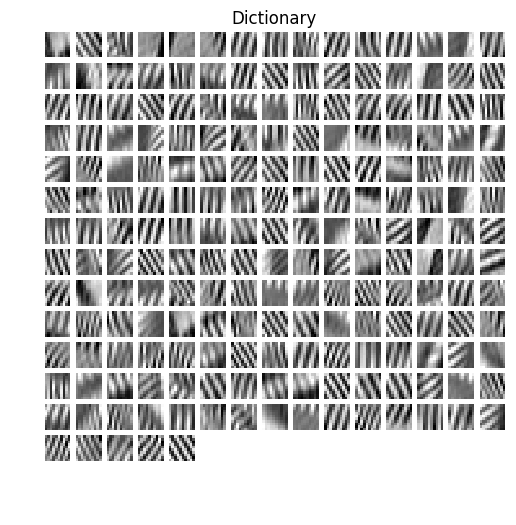# Dictionary Learning¶

Important: Please read the installation page for details about how to install the toolboxes. $\newcommand{\dotp}{\langle #1, #2 \rangle}$ $\newcommand{\enscond}{\lbrace #1, #2 \rbrace}$ $\newcommand{\pd}{ \frac{ \partial #1}{\partial #2} }$ $\newcommand{\umin}{\underset{#1}{\min}\;}$ $\newcommand{\umax}{\underset{#1}{\max}\;}$ $\newcommand{\umin}{\underset{#1}{\min}\;}$ $\newcommand{\uargmin}{\underset{#1}{argmin}\;}$ $\newcommand{\norm}{\|#1\|}$ $\newcommand{\abs}{\left|#1\right|}$ $\newcommand{\choice}{ \left\{ \begin{array}{l} #1 \end{array} \right. }$ $\newcommand{\pa}{\left(#1\right)}$ $\newcommand{\diag}{{diag}\left( #1 \right)}$ $\newcommand{\qandq}{\quad\text{and}\quad}$ $\newcommand{\qwhereq}{\quad\text{where}\quad}$ $\newcommand{\qifq}{ \quad \text{if} \quad }$ $\newcommand{\qarrq}{ \quad \Longrightarrow \quad }$ $\newcommand{\ZZ}{\mathbb{Z}}$ $\newcommand{\CC}{\mathbb{C}}$ $\newcommand{\RR}{\mathbb{R}}$ $\newcommand{\EE}{\mathbb{E}}$ $\newcommand{\Zz}{\mathcal{Z}}$ $\newcommand{\Ww}{\mathcal{W}}$ $\newcommand{\Vv}{\mathcal{V}}$ $\newcommand{\Nn}{\mathcal{N}}$ $\newcommand{\NN}{\mathcal{N}}$ $\newcommand{\Hh}{\mathcal{H}}$ $\newcommand{\Bb}{\mathcal{B}}$ $\newcommand{\Ee}{\mathcal{E}}$ $\newcommand{\Cc}{\mathcal{C}}$ $\newcommand{\Gg}{\mathcal{G}}$ $\newcommand{\Ss}{\mathcal{S}}$ $\newcommand{\Pp}{\mathcal{P}}$ $\newcommand{\Ff}{\mathcal{F}}$ $\newcommand{\Xx}{\mathcal{X}}$ $\newcommand{\Mm}{\mathcal{M}}$ $\newcommand{\Ii}{\mathcal{I}}$ $\newcommand{\Dd}{\mathcal{D}}$ $\newcommand{\Ll}{\mathcal{L}}$ $\newcommand{\Tt}{\mathcal{T}}$ $\newcommand{\si}{\sigma}$ $\newcommand{\al}{\alpha}$ $\newcommand{\la}{\lambda}$ $\newcommand{\ga}{\gamma}$ $\newcommand{\Ga}{\Gamma}$ $\newcommand{\La}{\Lambda}$ $\newcommand{\si}{\sigma}$ $\newcommand{\Si}{\Sigma}$ $\newcommand{\be}{\beta}$ $\newcommand{\de}{\delta}$ $\newcommand{\De}{\Delta}$ $\newcommand{\phi}{\varphi}$ $\newcommand{\th}{\theta}$ $\newcommand{\om}{\omega}$ $\newcommand{\Om}{\Omega}$

Instead of using a fixed data representation such as wavelets or Fourier, one can learn the representation (the dictionary) to optimize the sparsity of the representation for a large class of exemplar.

In :
%matplotlib inline

import matplotlib.pyplot as plt
import numpy as np

from nt_toolbox.signal import load_image, imageplot, plot_dictionary
from nt_toolbox.general import crop


## Dictionary Learning as a Non-convex Optimization Problem¶

Given a set $Y = (y_j)_{j=1}^m \in \RR^{n \times m}$ of $m$ signals $y_j \in \RR^m$, dictionary learning aims at finding the best dictionary $D=(d_i)_{i=1}^p$ of $p$ atoms $d_i \in \RR^n$ to sparse code all the data.

In this numerical tour, we consider an application to image denoising, so that each $y_j \in \RR^n$ is a patch of size $n=w \times w$ extracted from the noisy image.

The idea of learning dictionaries to sparse code image patch was first proposed in:

Olshausen BA, and Field DJ., <http://www.nature.com/nature/journal/v381/n6583/abs/381607a0.html Emergence of Simple-Cell Receptive Field Properties by Learning a Sparse Code for Natural Images.> Nature, 381: 607-609, 1996.

The sparse coding of a single data $y=y_j$ for some $j=1,\ldots,m$ is obtained by minimizing a $\ell^0$ constrained optimization $$\umin{ \norm{x}_0 \leq k } \frac{1}{2}\norm{y-Dx}^2 .$$ where the $\ell^0$ pseudo-norm of $x \in \RR^p$ is $$\norm{x}_0 = \abs{\enscond{i}{x(i) \neq 0}}.$$

The parameter $k>0$ controls the amount of sparsity.

Dictionary learning performs an optimization both on the dictionary $D$ and the set of coefficients $X = (x_j)_{j=1}^m \in \RR^{p \times m}$ where, for $j=1,\ldots,m$, $x_j$ is the set of coefficients of the data $y_j$. This joint optimization reads $$\umin{ D \in \Dd, X \in \Xx_k } E(X,D) = \frac{1}{2}\norm{Y-DX}^2 = \frac{1}{2} \sum_{j=1}^m \norm{y_j - D x_j}^2.$$

The constraint set on $D$ reads $$\Dd = \enscond{D \in \RR^{n \times p} }{ \forall i=1,\ldots,p, \quad \norm{D_{\cdot,i}} \leq 1 },$$ (the columns of the dictionary are unit normalized). The sparsity constraint set on $X$ reads $$\Xx_k = \enscond{X \in \RR^{p \times m}}{ \forall j, \: \norm{X_{\cdot,j}}_0 \leq k }.$$

We propose to use a block-coordinate descent method to minimize $E$: $$X^{(\ell+1)} \in \uargmin{X \in \Xx_k} E(X,D^{(\ell)}),$$ $$D^{(\ell+1)} \in \uargmin{D \in \Dd} E(X^{(\ell+1)},D).$$

One can show the convergence of this minimization scheme, see for instance

P. Tseng, <http://www.math.washington.edu/~tseng/papers/archive/bcr_jota.pdf Convergence of Block Coordinate Descent Method for Nondifferentiable Minimization>, J. Optim. Theory Appl., 109, 2001, 475-494.

We now define the parameter of the problem.

Width $w$ of the patches.

In :
w = 10


Dimension $n= w \times w$ of the data to be sparse coded.

In :
n = w*w


Number of atoms $p$ in the dictionary.

In :
p = 2*n


Number $m$ of patches used for the training.

In :
m = 20*p


Target sparsity $k$.

In :
k = 4


## Patch Extraction¶

Since the learning is computationnaly intensive, one can only apply it to small patches extracted from an image.

In :
f = crop(load_image("nt_toolbox/data/barb.bmp"), 256)
n0 = f.shape


Display the input image.

In :
plt.figure(figsize = (5,5))
imageplot(f)Random patch location.

In :
q = 3*m

# Random sampling of coordinates of the top left corner or the patches
x = (np.random.random((1, 1, q))*(n0-w)).astype(int)
y = (np.random.random((1, 1, q))*(n0-w)).astype(int)


Extract lots of patches $y_j \in \RR^n$, and store them in a matrix $Y=(y_j)_{j=1}^m$.

In :
[dY, dX] = np.meshgrid(range(w), range(w))
dX = np.tile(dX, (q, 1, 1)).transpose((1, 2, 0))
dY = np.tile(dY, (q, 1, 1)).transpose((1, 2, 0))
Xp = np.tile(x, (w, w, 1)) + dX
Yp = dY + np.tile(y, (w, w, 1))

# Extract patches
Y = f.flatten()[Yp+Xp*n0]
Y = Y.reshape((w*w, q))


We remove the mean, since we are going to learn a dictionary of zero-mean and unit norm atom.

In :
Y -= Y.mean(axis=0)


Only keep those with largest energy.

In :
energies = np.sum(Y**2, axis=0)
indexes = np.argsort(energies)[::-1]
Y = Y[:, indexes[:m]];


We consider a dictionary $D \in \RR^{n \times p}$ of $p \geq n$ atoms in $\RR^n$. The initial dictionary $D$ is computed by a random selection of patches, and we normalize them to be unit-norm.

In :
def projC(Y):
''' Scale the patches to unit norm '''
norm = np.tile(np.linalg.norm(Y, axis=0), (Y.shape, 1))
Y = np.divide(Y, norm)
return  Y

# Pick p indexes at random to pick patches from Y
sel = np.random.permutation(range(m))[:p]
D = projC(Y[:, sel])
D0 = D.copy()


Display the initial dictionary.

In :
plt.figure(figsize=(10,6))
plot_dictionary(D)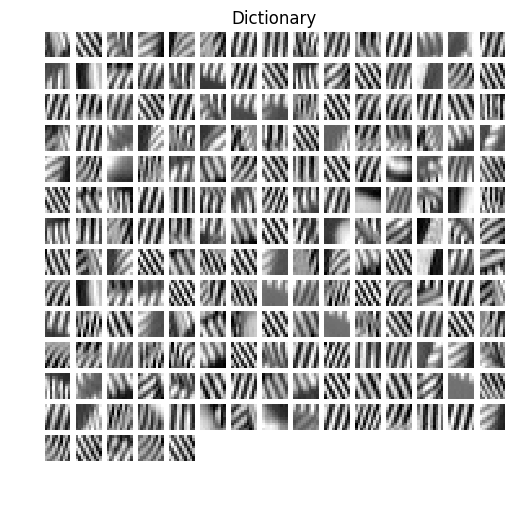## Update of the Coefficients $X$¶

The optimization on the coefficients $X$ requires, for each $y_j = Y_{\cdot,j}$ to compute $x_j = X_{\cdot,j}$ that solves $$\umin{ \norm{x_j}_0 \leq k } \frac{1}{2} \norm{y-D x_j}^2.$$

This is a non-smooth and non-convex minimization, that can be shown to be NP-hard. A heuristic to solve this method is to compute a stationary point of the energy using the Foward-Backward iterative scheme (projected gradient descent): $$x_j \leftarrow \text{Proj}_{\Xx_k}\pa{ x_j - \tau D^* ( D x_j - y ) } \qwhereq \tau < \frac{2}{\norm{D D^*}}.$$

Denoting $\abs{\bar x(1)} \leq \ldots \leq \abs{\bar x(n)}$ the ordered magnitudes of a vector $x \in \RR^n$, the orthogonal projector on $\Xx_k$ reads $z = \text{Proj}_{\Xx_k}(x)$ with $$\forall i=1,\ldots,n, \quad z(i) = \choice{ x(i) \qifq \abs{x(i)} \geq \abs{\bar x(k)}, \\ z(i) = 0 \quad \text{otherwise}. }$$

In :
def projX(X, k):
''' Sparsity projection, keeps the k largest coefficients '''
X = X * (abs(X) >= np.sort(abs(X), axis=0)[-k, :])
return X


Exercise 1

Perform the iterative hard thresholding, and display the decay of the energy $J(x_j) = \norm{y_j-D x_j}^2$ for several $j$. Remark: note that the iteration can be performed in parallel on all $x_j$.

In :
run -i nt_solutions/sparsity_4_dictionary_learning/exo1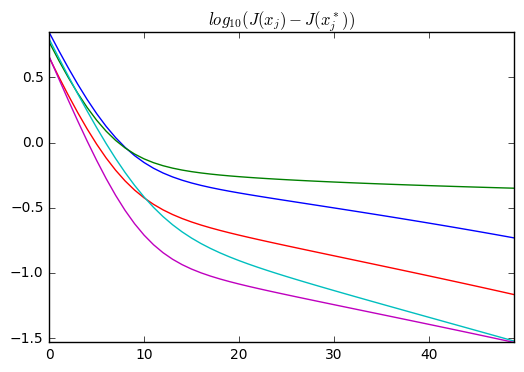In :
## Insert your code here.


## Update the Dictionary $D$¶

Once the sparse coefficients $X$ have been computed, one can udpate the dictionary. This is achieve by performing the minimization $$\umin{D \in \Dd} \frac{1}{2}\norm{Y-D X}^2.$$

One can perform this minimization with a projected gradient descent $$D \leftarrow \text{Proj}_{\Cc}\pa{ D - \tau (DX - Y)X^* }$$ where $\tau < 2/\norm{XX^*}.$

Note that the orthogonal projector $\text{Proj}_{\Cc}$ is implemented in the function |ProjC| already defined.

Exercise 2

Perform this gradient descent, and monitor the decay of the energy.

In :
run -i nt_solutions/sparsity_4_dictionary_learning/exo2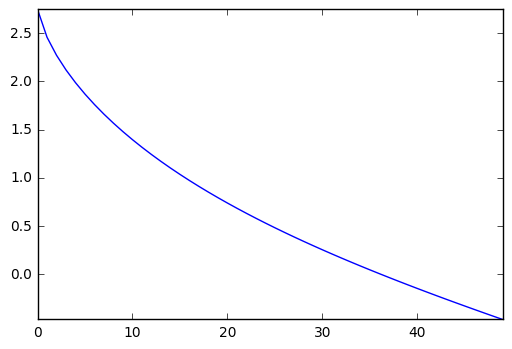In :
## Insert your code here.


Exercise 3

Perform the dictionary learning by iterating between sparse coding and dictionary update.

In :
run -i nt_solutions/sparsity_4_dictionary_learning/exo3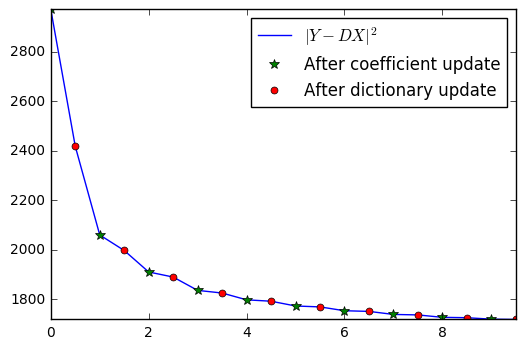In :
## Insert your code here.


Display the dictionary.

In :
plt.figure(figsize=(10,6))
plot_dictionary(D)